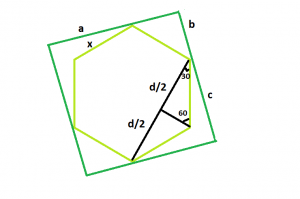# Largest hexagon that can be inscribed within a square

• Difficulty Level : Hard
• Last Updated : 25 Jun, 2022

Given side of a square a, the task is to find the side of the largest hexagon that can be inscribed within the given square.
Examples:

Input: a = 6
Output: 3.1056
Input: a = 8
Output: 4.1408Approach:: Let, the side of the hexagon be x and assume that the side of the square, a gets divided into smaller length b & bigger length c i.e. a = b + c
Now from the figure, we see,

b2 + b2 = x2 which gives b = x / √2
Now again, d / (2 * x) = cos(30) = √3 / 2 i.e. d = x√3
And, c2 + c2 = d2 which gives c = d / √2 = x√3 / √2
Since, a = b + c. So, a = x / √2 + x√3 / √2 = ((1 + √3) / √2) * x = 1.932 * x
So, side of the hexagon, x = 0.5176 * a

Below is the implementation of the above approach:

## C++

 `// C++ Program to find the biggest hexagon which``// can be inscribed within the given square``#include ``using` `namespace` `std;` `// Function to return the side``// of the hexagon``float` `hexagonside(``float` `a)``{` `    ``// Side cannot be negative``    ``if` `(a < 0)``        ``return` `-1;` `    ``// Side of the hexagon``    ``float` `x = 0.5176 * a;``    ``return` `x;``}` `// Driver code``int` `main()``{``    ``float` `a = 6;``    ``cout << hexagonside(a) << endl;``    ``return` `0;``}`

## Java

 `// Java  Program to find the biggest hexagon which``// can be inscribed within the given square` `import` `java.io.*;` `class` `GFG {``    ` `// Function to return the side``// of the hexagon``static` `double` `hexagonside(``double` `a)``{` `    ``// Side cannot be negative``    ``if` `(a < ``0``)``        ``return` `-``1``;` `    ``// Side of the hexagon``    ``double` `x = (``0.5176` `* a);``    ``return` `x;``}` `// Driver code``    ``public` `static` `void` `main (String[] args) {` `        ``double` `a = ``6``;``        ``System.out.println (hexagonside(a));``    ``}``//This code is contributed by ajit.   ``}`

## Python 3

 `# Python 3 Program to find the biggest``# hexagon which can be inscribed within``# the given square` `# Function to return the side``# of the hexagon``def` `hexagonside(a):` `    ``# Side cannot be negative``    ``if` `(a < ``0``):``        ``return` `-``1``;` `    ``# Side of the hexagon``    ``x ``=` `0.5176` `*` `a;``    ``return` `x;` `# Driver code``a ``=` `6``;``print``(hexagonside(a));` `# This code is contributed``# by Akanksha Rai`

## C#

 `// C# Program to find the biggest hexagon which``// can be inscribed within the given square``using` `System;` `class` `GFG``{``        ` `// Function to return the side``// of the hexagon``static` `double` `hexagonside(``double` `a)``{` `    ``// Side cannot be negative``    ``if` `(a < 0)``        ``return` `-1;` `    ``// Side of the hexagon``    ``double` `x = (0.5176 * a);``    ``return` `x;``}` `// Driver code``public` `static` `void` `Main ()``{``    ``double` `a = 6;``    ``Console.WriteLine(hexagonside(a));``}``}` `// This code is contributed by Ryuga.`

## PHP

 ``

## Javascript

 ``

Output:

`3.1056`

Time Complexity: O(1)

Auxiliary Space: O(1)

My Personal Notes arrow_drop_up